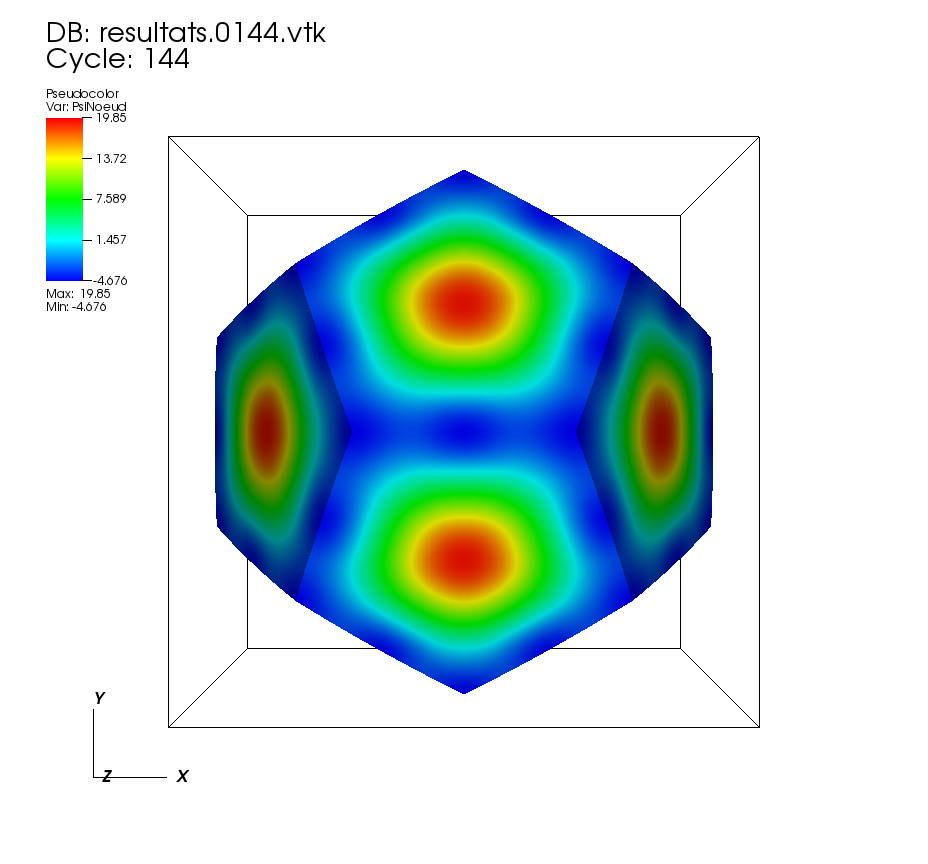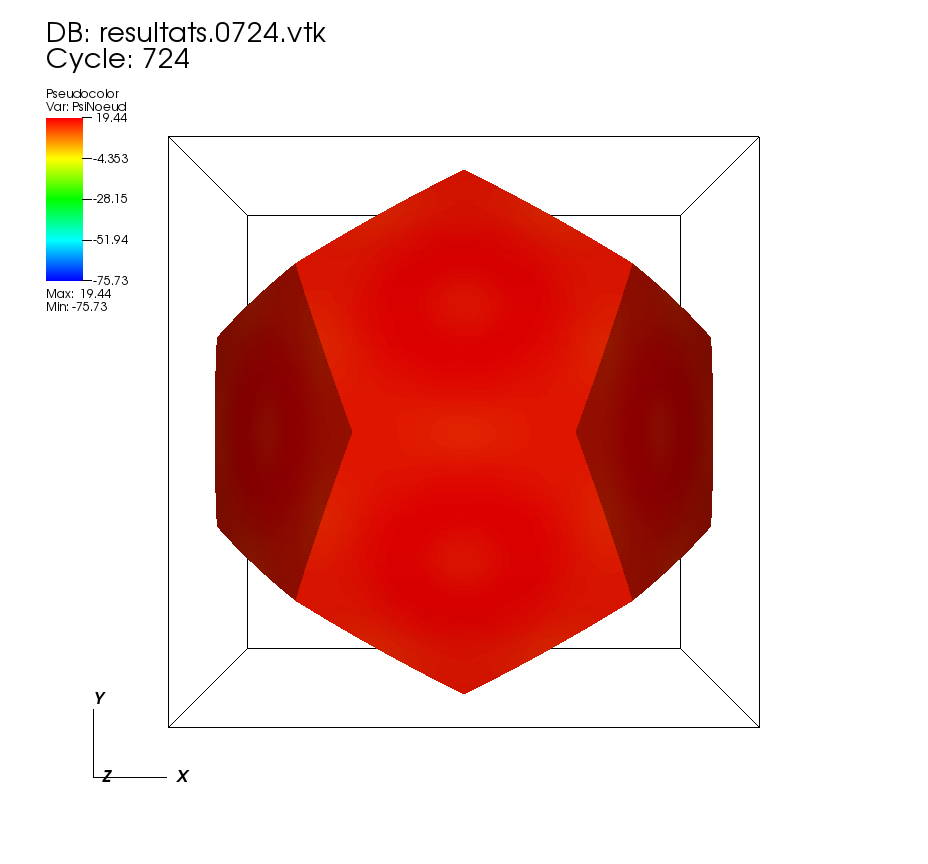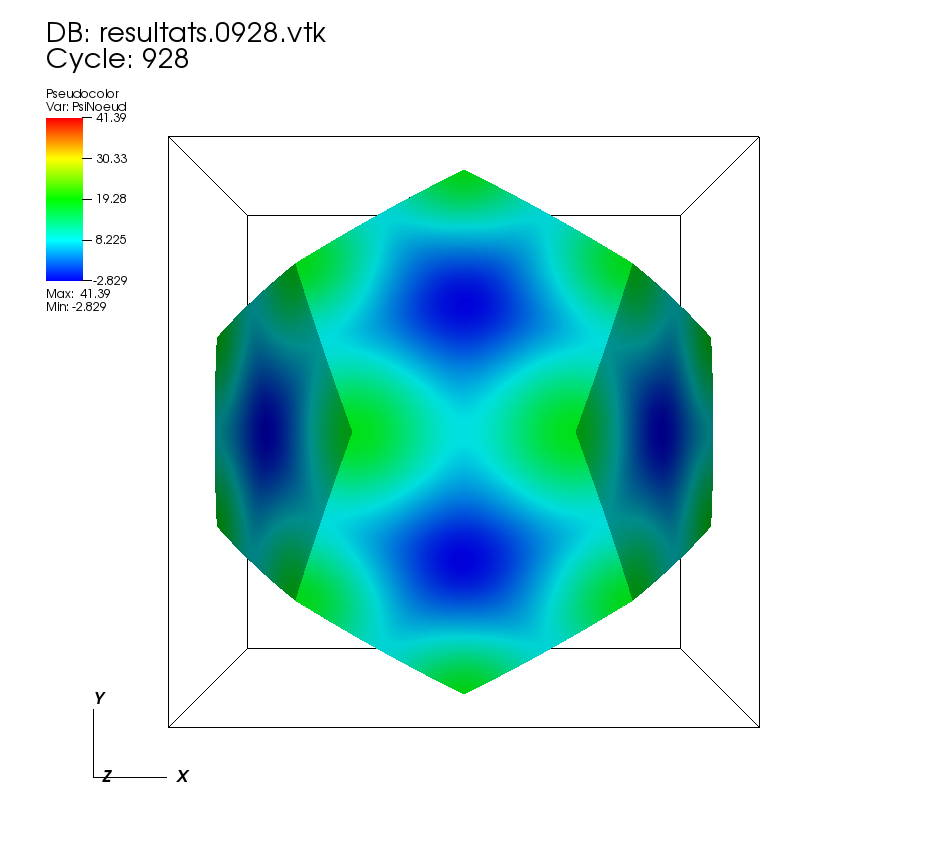##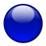Résolution numérique de l'équation des ondes sur l'espace dodécaédrique de Poincaré.

(Wave Computation on the Poincaré dodecahedral space. Class. Quantum Grav. 30 (2013) 235010.)

The Poincaré dodecahedral space (PDS) is a plausible multi-connected universe model with constant positive spatial curvature.
This 3-manifold without boundary is the quotient of the unit 3-sphere S3 under the action of the binary icosahedral group I∗ of isometries of S3, with I∗ acting by left multiplication.
PDS is also represent by a fundamental domain F ⊂ S3 and an equivalence relation ∼ such that S3/I∗ = F/ ∼ .
F is a regular spherical dodecahedron (dual of a regular icosahedron), and ∼ is obtained by identifying the opposite pentagonal faces of F after rotating by π/5 in the clockwise direction around the axis orthogonal to the face. 120 such spherical dodecahedra tile the 3-sphere in the pattern of a regular 120-cell.

This is the diagramm of vertices of F, and a diagramm of the vertices of the 120-cell. Staight lines are drawn to better see the pentagons.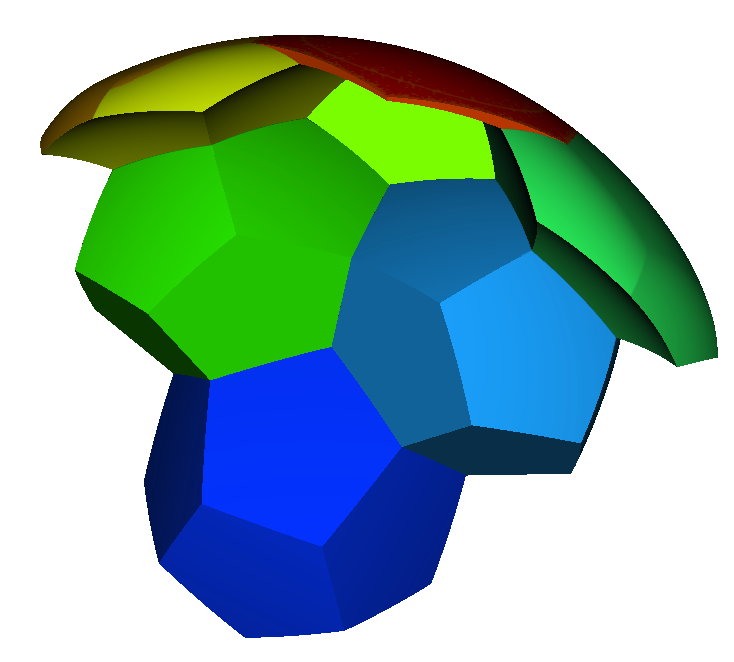In fact PDS is endowed with the spherical metric induced by this one of S3, and  this picture is a visualisation of a part of S3 tilled by the 120 dodecahedra.
That is you can see a projection in R3 of a fundamental domain (dark blue) and six of its image by elements of the binary icosahedral group.

### Then, we compute the solutions of the wave equation in the time domain, by using a variational method and a discretization with finite elements. There are few movies of solutions:

Time evolution with a centered initial data:
 On some faces:  •t=0 to t=1 On a cutting plane x=0:   •t=0 to t=1

Time evolution with a non centered initial data:
 On some faces:  • t=0 to t=0.5   •t=0.5 to t=1 On other faces:  •t=0 to t=0.5   • t=0.5 to t=1 On a cutting plane z=0:  • t=0 to t=0.5  •t=0.5 to t=1 On a cutting plane x=0:  •t=0 to 0.5  • t=0.5 to t=1.

Time evolution with another initial data:
 On some faces:  • t=0 to t=5   •t=5 to t=10 On a cutting plane:  • t=0 to t=5  •t=5 to t=10 On two cutting planes:  •t=0 to 0.5

The support of the two first initial data is far from $\partial \mathcal{F}_v$, therefore they respect obviously the constraint of the equivalent points. It is interesting to consider the last initial data involving several equivalent points.

### Here are some characteristic pictures for a centered initial data. At each time there are a view of the solution on a fundamental domain: